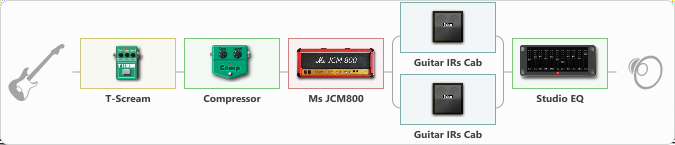# Ac Dc

Discussion in 'ToneLib-GFX presets' started by Danil_pr, Jul 31, 2021.

1. Ac Dc

Preset name: Ac Dc

Effects chain:Effect: "T-Scream" (Overdrive / Distortion), active - "yes"
{
"Drive" = 23
"Tone" = 54
"Level" = 43
}

Effect: "Compressor" (Dynamics / Filter), active - "yes"
{
"Sense" = 21
"Level" = 50
}

Effect: "Ms JCM800" (Amp simulators), active - "yes"
{
"Gain" = 35
"Bass" = 77
"Middle" = 71
"Treble" = 35
"Presence" = 68
"Master" = 100
"Level (dB)" = 0
}

Effect: "Splitter" (Dynamics / Filter)
{
"A-Bypass" = Off
"A-Pan" = 0
"A-Level" = 55
"B-Bypass" = Off
"B-Pan" = 0
"B-Level" = 55

'A' branch:
{

Effect: "Guitar IRs Cab" (Cabinets), active - "yes"
{
"Model" = Marshall 1960b (4x12")
"Mic Position" = Center
"Mic Distance" = Near
"Low Cut (Hz)" = 60
"Hi Cut (kHz)" = 9.0
"Mix" = 100
"Level (dB)" = 0
}
}
'B' branch:
{

Effect: "Guitar IRs Cab" (Cabinets), active - "yes"
{
"Model" = Marshall 1960b (4x12")
"Mic Position" = Center
"Mic Distance" = Middle
"Low Cut (Hz)" = 60
"Hi Cut (kHz)" = 9.0
"Mix" = 100
"Level (dB)" = 0
}
}
}

Effect: "Studio EQ" (Dynamics / Filter), active - "yes"
{
"31 Hz" = -2
"62 Hz" = 4
"125 Hz" = 4
"250 Hz" = 5
"500 Hz" = -5
"1 kHz" = 2
"2 kHz" = -2
"4 kHz" = 4
"8 kHz" = 6
"16 kHz" = 0
"Level (dB)" = 0
}

Note: You will need to download and install the ToneLib-GFX software to use the preset.

File size:
745 bytes
Views:
5,761
2. nice